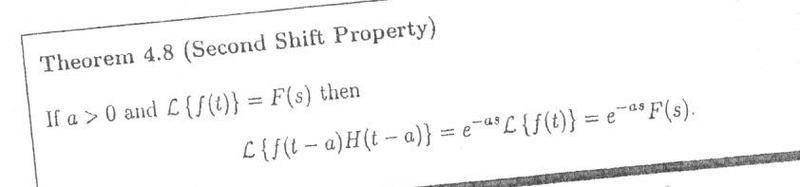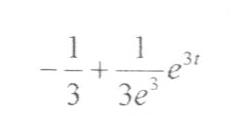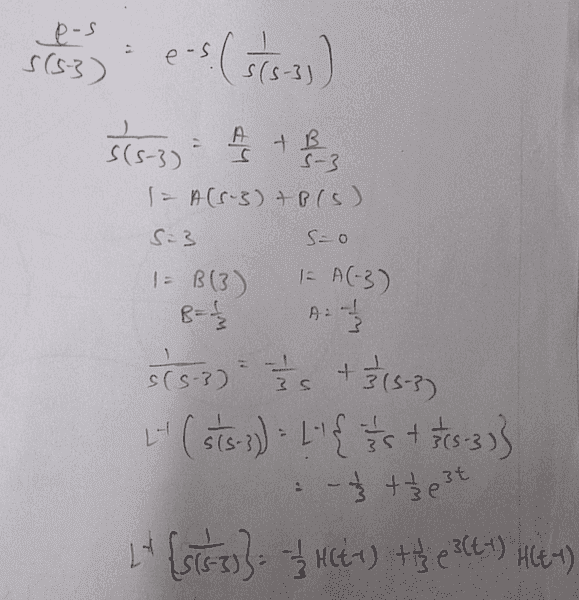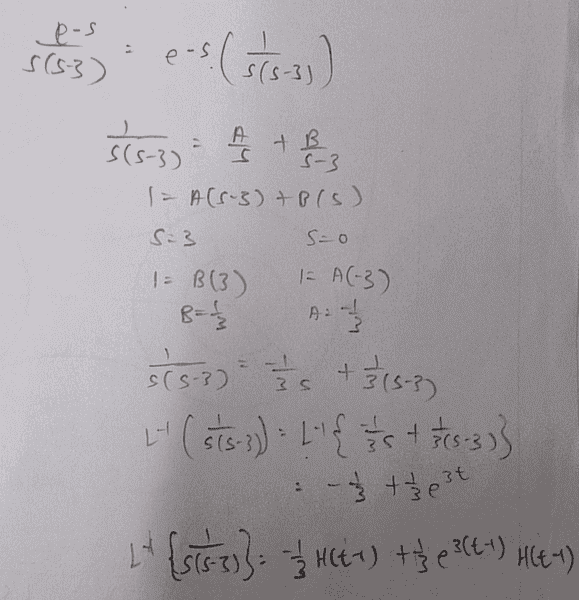# Laplace transform of (e^-s) / [ (s)(s-3) ]

• foo9008

## Homework Statement

find the laplace transform of (e^-s) / [ (s)(s-3) ]

since there's (e^-s) which can be found in L { f(t-a) H(t-a) } = (e^-(as)) F(s) , so , i found a = 1 , then i found F(s) = 1/ [ (s)(s-3) ] , formula :i have attached the working below , is it correct ? btw , the author's ans doesn't have H(t-a) , is the author's ans wrong ?
author's ans :## The Attempt at a Solution

my working :which is correct ? the author ? or me ?

Last edited:

## Homework Statement

find the laplace transform of (e^-s) / [ (s)(s-3) ]

since there's (e^-s) which can be found in L { f(t-a) H(t-a) } = (e^-(as)) F(s) , so , i found a = 1 , then i found F(s) = 1/ [ (s)(s-3) ] , formula :i have attached the working below , is it correct ? btw , the author's ans doesn't have H(t-a) , is the author's ans wrong ?
author's ans :## The Attempt at a Solution

my working :which is correct ? the author ? or me ?

There is no way that an author would have asked you to find the Laplace transform of ##e^{-s}/(s(s-3))##. Are you sure it does not ask for the INVERSE Laplace transform? There is a huge difference between these two concepts.